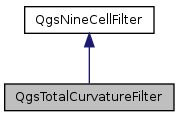QGIS API Documentation  2.0.1-Dufour
QgsTotalCurvatureFilter Class Reference

Calculates total curvature as described by Wilson, Gallant (2000): terrain analysis. More...

`#include <qgstotalcurvaturefilter.h>`

Inheritance diagram for QgsTotalCurvatureFilter:[legend]
Collaboration diagram for QgsTotalCurvatureFilter:[legend]

## Public Member Functions

QgsTotalCurvatureFilter (const QString &inputFile, const QString &outputFile, const QString &outputFormat)
~QgsTotalCurvatureFilter ()Public Member Functions inherited from QgsNineCellFilter
QgsNineCellFilter (const QString &inputFile, const QString &outputFile, const QString &outputFormat)
Constructor that takes input file, output file and output format (GDAL string)
virtual ~QgsNineCellFilter ()
int processRaster (QProgressDialog *p)
Starts the calculation, reads from mInputFile and stores the result in mOutputFile.
double cellSizeX () const
void setCellSizeX (double size)
double cellSizeY () const
void setCellSizeY (double size)
double zFactor () const
void setZFactor (double factor)
double inputNodataValue () const
void setInputNodataValue (double value)
double outputNodataValue () const
void setOutputNodataValue (double value)

## Protected Member Functions

float processNineCellWindow (float *x11, float *x21, float *x31, float *x12, float *x22, float *x32, float *x13, float *x23, float *x33)
Calculates total curvature from nine input values.Protected Attributes inherited from QgsNineCellFilter
QString mInputFile
QString mOutputFile
QString mOutputFormat
double mCellSizeX
double mCellSizeY
float mInputNodataValue
The nodata value of the input layer.
float mOutputNodataValue
The nodata value of the output layer.
double mZFactor
Scale factor for z-value if x-/y- units are different to z-units (111120 for degree->meters and 370400 for degree->feet)

## Detailed Description

Calculates total curvature as described by Wilson, Gallant (2000): terrain analysis.

Definition at line 24 of file qgstotalcurvaturefilter.h.

## Constructor & Destructor Documentation

 QgsTotalCurvatureFilter::QgsTotalCurvatureFilter ( const QString & inputFile, const QString & outputFile, const QString & outputFormat )

Definition at line 20 of file qgstotalcurvaturefilter.cpp.

 QgsTotalCurvatureFilter::~QgsTotalCurvatureFilter ( )

Definition at line 26 of file qgstotalcurvaturefilter.cpp.

## Member Function Documentation

 float QgsTotalCurvatureFilter::processNineCellWindow ( float * x11, float * x21, float * x31, float * x12, float * x22, float * x32, float * x13, float * x23, float * x33 )
protectedvirtual

Calculates total curvature from nine input values.

The input values and the output value can be equal to the nodata value if not present or outside of the border. Must be implemented by subclasses

Implements QgsNineCellFilter.

Definition at line 31 of file qgstotalcurvaturefilter.cpp.

The documentation for this class was generated from the following files: